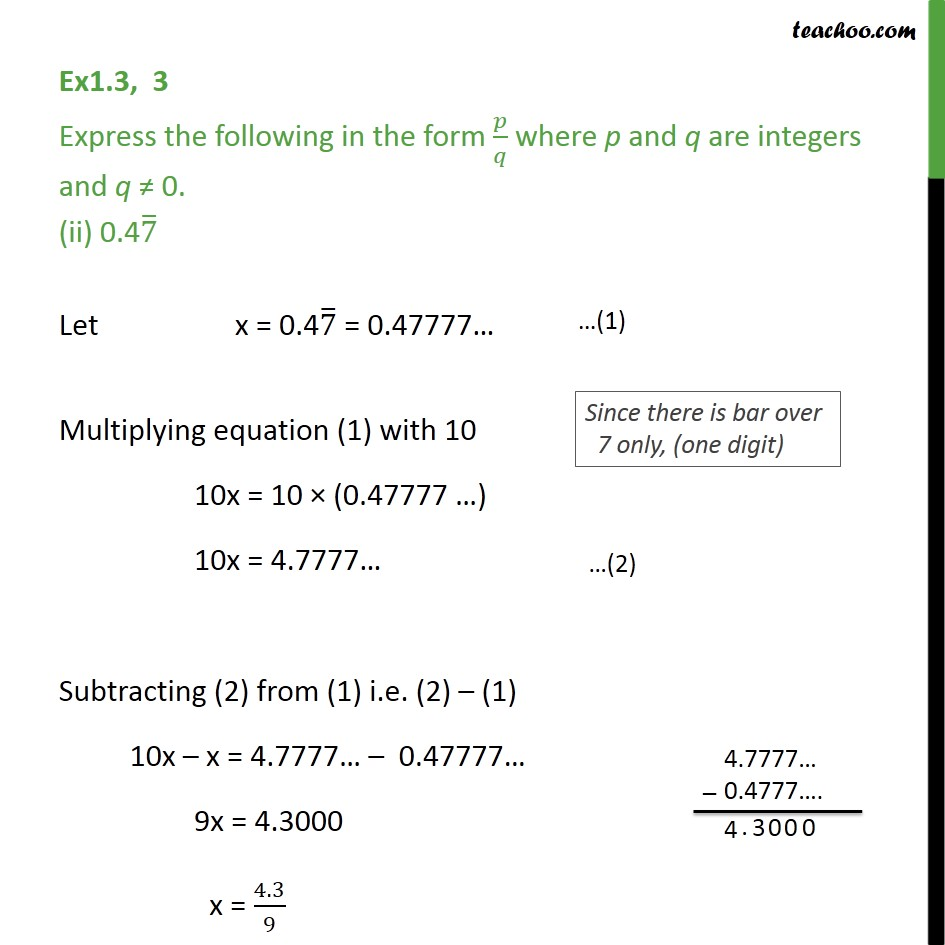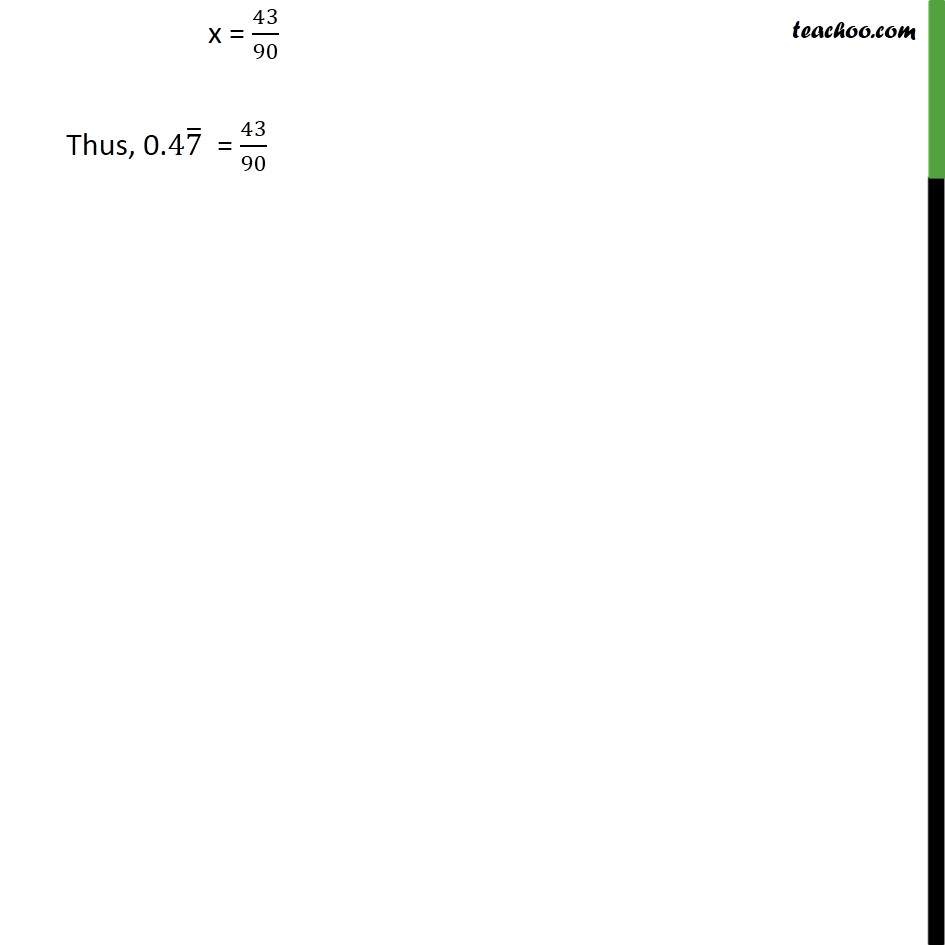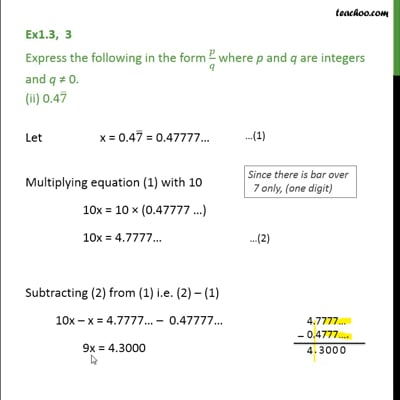Ex 1.3

Chapter 1 Class 9 Number Systems
Serial order wiseThis video is only available for Teachoo black users

Maths Crash Course - Live lectures + all videos + Real time Doubt solving!

### Transcript

Ex1.3, 3 Express the following in the form 𝑝/𝑞 where p and q are integers and q ≠ 0. (ii) 0.47 ̅ Let x = 0.47 ̅ = 0.47777… Multiplying equation (1) with 10 10x = 10 × (0.47777 …) 10x = 4.7777… Subtracting (2) from (1) i.e. (2) – (1) 10x – x = 4.7777… – 0.47777… 9x = 4.3000 x = 4.3/9 x = 43/90 Thus, 0.47 ̅ = 43/90